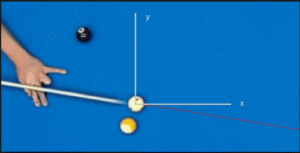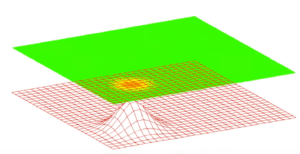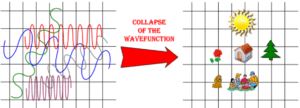Home » quantum mechanics

# quantum mechanics

« Back to Glossary Index

In quantum mechanics, physicists study how tiny particles behave. These include atoms, the components of atoms like electrons and protons, and other tiny particles like photons of light. These tiny particles follow the laws of quantum mechanics and are called “quantum particles.”Carbon molecules emitting a photon, a tiny particle of light (in green). Yes, the photon looks like it’s moving towards the molecules—no accounting for the whims of artists. [Image source: Nancy Ambrosiano, Los Alamos National Laboratory, July 2017 News Release, “Single-photon emitter has promise for quantum info-processing,” (Public domain)https://www.lanl.gov/discover/news-release-archive/2017/July/0731-single-photon-emitter.php]Billiard balls epitomize objects that follow Newton’s Laws of Motion.

In the early 1900’s, physicists started experimenting with atoms and molecules. They discovered that atoms and their components follow different laws from those of ordinary objects like tables and chairs, so-called “macroscopic” objects.

The mathematical laws governing the movements and forces among tables and chairs, “classical mechanics,” were first articulated by Isaac Newton in the 1600’s. For example, Newton developed the mathematical law of the amount of force exerted by a chair when thrown at a  table: Force = Mass times Acceleration.

However, when physicists of the early 1900’s used the equations of classical mechanics to predict the results of experiments with electrons and tiny particles of light, their predictions turned out to be erroneous. After considerable dismay, confusion, and fumbling around, they came up with quantum theory. By the 1920’s and 30’s, they were able to articulate the laws of quantum theory with mathematical equations. The mathematical version of this field then became known as “quantum mechanics” on analogy with “classical mechanics.”

Quantum mechanics is also often called “quantum physics.” a term which doesn’t emphasize the mathematical aspect of the field. The term “quantum physics” can also be applied to the early years before the mathematical equations of quantum mechanics were developed.The quantum sublevel of reality appears a red mesh wave. It forms a particle in our macroscopic level of reality (orange/yellow dot). [Image source: stills from Fermilab video by Dr. Don Lincoln, “Quantum Field Theory” (in the public domain) Jan. 14, 2016; https://www.youtube.com/watch?v=FBeALt3rxEA&feature=youtu.be.]

Quantum mechanics describes a different reality from the one that we experience daily. It’s a sublevel of reality that co-exists with ours and underlies it. I’ll call it “Quantumland.” In Quantumland,1"Quantumland" is a term coined by Dr. Ruth E. Kastner, one of the developers of the Transactional Interpretation of quantum mechanics. Ruth E. Kastner, Understanding Our Unseen Reality, Solving Quantum an electron is a “wave” that instantaneously turns into a particle the moment that we detect it. “Wave” is in quotes because it’s not a wave like a water wave or other waves that we encounter at the macroscopic level. The mathematical description of a quantum wave is quite different from that of a water wave. The mathematical equations that describe quantum particles don’t fit how we think mathematics should describe things in the physical universe.2The mathematical description of a quantum wave is called a "wave function." A wave function can include imaginary numbers. Imaginary numbers are based on the square root of negative 1.

## QuantumlandQuantumland (left) and macroscopic world of objects that we perceive (right). The transformation from the wavy quantum state to particles that we detect is called the “Collapse of the Wave Function.”  [Image source: David Chalmers and Kelvin McQueen, “Consciousness and the Collapse of the Wave Function” http://consc.net/slides/collapse.pdf

In Quantumland,1"Quantumland" is a term coined by Dr. Ruth E. Kastner, one of the developers of the Transactional Interpretation of quantum mechanics. Ruth E. Kastner, Understanding Our Unseen Reality, Solving Quantum quantum particles behave in a number of odd ways:

• As mentioned, electrons, photons, and other quantum particles can act like a kind of wave at one moment and a particle the next. This transition is demonstrated in the above video.
• Quantum particles, in certain regards, appear to behave with true randomness. The properties of individual particles, such as their position when detected, do not appear to be determined by causal events. However, the probability that a collection of particles will manifest particular properties can be predicted. For example, the percentage of particles that will be detected in particular positions can be predicted.
• Some of the properties of quantum particles, such as momentum, are not defined until the particles interact with some other part of the physical universe. For example, the direction of travel of a photon of light appears to be many directions at the same time, as if smelling all the possibilities. When detected, that is, absorbed by an electron, the photon will be found in a definite position, one in accord with the straight-line travel.
• Quantum particles appear to cause effects prior in time (retro-causality).
• Quantum particles such as electrons can disappear from one side of a barrier and instantaneously appear on the other. This, despite having insufficient energy for this maneuver and without actually passing through the barrier. This is “quantum tunneling.”
• Particles which have interacted together are “entangled.” The behaviors of entangled particles are correlated despite any amount of distance separating them. For example, two entangled electrons sitting halfway across the universe from each other will always coordinate their spins. If one begins to spin clockwise, the other will begin to spin counterclockwise. According to the Theory of Special Relativity, which prohibits faster than light communication, this cannot be due to signaling each other. It appears to be due to a simultaneity of behavior which physicists can describe but not explain.
• Particles, known as “virtual particles,” can appear with no material source nor energy source and disappear almost instantaneously. These particles might be thought to violate the Law of Conservation of Energy. But the violation occurs so briefly that the mathematics of quantum mechanics, specifically, the Heisenberg Uncertainty Principle, is adequate to describe this behavior.

## Interpretations of Quantum Mechanics

Most physicists agree on the key mathematical equations of quantum mechanics. But physicists have not reached agreement on the implications of quantum mechanics for the nature of quantum particles and the nature of reality. Physicists have developed about 15 different interpretations which assign meaning to quantum physics, describing both how quantum particles behave and the nature of reality.

*Quantumland is a term coined by Dr. Ruth E. Kastner, one of the developers of the Transactional Interpretation of quantum mechanics. Ruth E. Kastner, Understanding Our Unseen Reality, Solving Quantum Riddles; Imperial College Press, 2015, London.

**The mathematical description of a quantum wave is called a “wave function.” A wave function can include imaginary numbers. Imaginary numbers are based on the square root of negative 1. You are right if you’re wondering what number squared (multiplied times itself) equals negative 1. There is no such number. The square root of negative 1 does not exist and is called an “imaginary number.” Nevertheless, imaginary numbers are needed when describing quantum waves. This is only one of the issues that besets our ability to conceptualize the mathematics of quantum waves and to consider these waves real. Another issue is that the quantum wave describes probabilities as to how the particle will behave rather than describing definite properties.

FOOTNOTES
1"Quantumland" is a term coined by Dr. Ruth E. Kastner, one of the developers of the Transactional Interpretation of quantum mechanics. Ruth E. Kastner, Understanding Our Unseen Reality, Solving Quantum Riddles; Imperial College Press, 2015, London.
2The mathematical description of a quantum wave is called a "wave function." A wave function can include imaginary numbers. Imaginary numbers are based on the square root of negative 1. You are right if you're wondering what number squared (multiplied times itself) equals negative 1. There is no such number. The square root of negative 1 does not exist and is called an "imaginary number." Nevertheless, imaginary numbers are needed when describing quantum waves. This is only one of the issues that besets our ability to conceptualize the mathematics of quantum waves and to consider these waves real. Another issue is that the quantum wave describes probabilities as to how the particle will behave rather than describing definite properties.

This site uses Akismet to reduce spam. Learn how your comment data is processed.